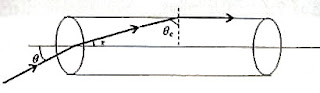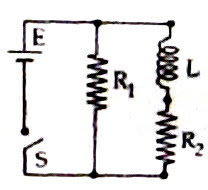## Wednesday, May 27, 2009

### AIEEE 2009 Multiple Choice Questions on Optics

I believe in standardising automobiles, not human beings

– Albert Einstein

The following three questions were included from optics in the All India Engineering/Architecture Entrance Examination (AIEEE) 2009 question paper:(1) A transparent solid cylindrical rod has a refractive index of 2/√3. It is surrounded by air. A light ray is incident at the mid point of one end of the rod as shown in the figure. The incident angle θ for which the light ray grazes along the wall of the rod is

(1) sin–1(1/√3)

(2) sin–1(1/2)

(3) sin–1(√3/2)

(4) sin–1(2/√3)

If n is the refractive index and θc is the critical angle of the material of the rod, we have n = 1/sinθc so that

sinθc = 1/n = √3/2.If r represents the angle of refracion (as shown in the figure), we have

1×sinθ = nsin r = (2/√3) ×sin(90º θc)

Or, sinθ = (2/√3) ×cos θc

But cos θc = ½ since sin θc = √3/2

[You may imagine a right angled triangle with opposite side √3 and hypotenuse 2. The adjacent side will be 1]

Substituting for cos θc, we obtain

sinθ = (2/√3) × ½ = 1/√3 so that θ = sin–1(1/√3)

(2) In an optics experiment, with the position of the object fixed, a student varies the position of a convex lens and for each position, the screen is adjusted to get a clear image of the object. A graph between the object distance u and the image distance v, from the lens, is plotted using the same scale for the two axes. A straight line passing through the origin and making an angle of 45° with the x-axis meets the experimental curve at P. The coordinates of P will be

(1) (4f, 4f)

(2) (2f, 2f)

(3) (f/2, f/2)

(4) (f, f)

This is a well known experiment which most of you might have performed. As such most of you know the answer: (2f, 2f)

We have 1/v – 1/u = 1/f

Applying the Cartesian sign convention, v is positive and u is negative so that

1/v + 1/u = 1/f

Since the straight line makes an angle of 45º, we have v = u at P and each must be equal to 2f to satisfy the equation, 1/v + 1/u = 1/f

(3) A mixture of light, consisting of wavelength 590 nm and an unknown wavelength, illuminates Young’s double slit and gives rise to two overlapping interference patterns on the screen. The central maximum of both lights coincide. Further, it is observed that the third bright fringe of known light coincides with the 4th bright fringe of the unknown light. From this data, the wavelength of the unknown light is

(1) 776.8 nm

(2) 393.4 nm

(3) 885.0 nm

(4) 442.5 nm

The fringe width β is given by

β = λD/d where λ is the wave length of light, D is the distance between the double slit and the screen and d is the double slit separation.

Since three fringes due to the light of wave length 590 nm exactly occupy the space occupied by four fringes due to the unknown light, we can write

3×590×D/d = 4λD/d where λ is the wave length of the unknown light (in nanometer).

This gives λ = 442.5 nm

You will find additional multiple choice questions (with solution) at the following locations:

1. apphysicsresources(i)

## Monday, May 11, 2009

### Kerala Engineering Entrance (KEAM) 2008 Questions on Nuclear Physics

Science without religion is lame, religion without science is blind.

– Albert Einstein

KEAM Engineering 2009 Exam will begin on 25th of this month. Here are the questions on nuclear physics which appeared in last year’s physics eam:

(1) The nuclear radius of a certain nucleus is 7.2 fm and it has a charge of 1.28×10–17C.The number of neutrons inside the nucleus is

(a) 136

(b) 142

(c) 140

(d) 132

(e) 126

The nuclear radius R is related to the mass number A as

R = 1.2 A1/3

On substituting for R in this equation we obtain A1/3 = 6 so that A = 216.

Since the total charge of the nucleus (carried by all the protons) is given as 1.28×10–17 coulomb and the charge on a proton is 1.6×10–19 coulomb, the number of protons (Z) in the nucleus is (1.28×10–17) /(1.6×10–19) = 80.

Therefore, the number of neutrons in the nucleus, N = A Z = 216 – 80 = 136.

(2) The energy released in the fission of 1 kg of 92U235 is (Energy per fission = 200 MeV)

(a) 5.1×1026 eV

(b) 5.1×1026 J

(c) 8.2×1013 J

(e) 5.1×1023 MeV

1 kg of 92U235 contains 1/0.235 moles and each mole contains 6.02×1023 atoms. Therefore1 kg of 92U235 contains (6.02×1023)/ 0.235 atoms.

Therefore, the energy E released in the fission of 1 kg of 92U235 is given by

E = (6.02×1023) ×200 / 0.235 MeV = 5.1×1026 MeV = 5.1×1032 eV

Since 1 eV = 1.6×10–19 joule, the above energy is (5.1×10 32)×(1.6×10–19) joule. This works out to approximately 8.2×1013 J.

(3) Which one of the following statements is true if half life of a radioactive substance is 1 month?

(a) 7/8th part of the substance will disintegrate in 3 months

(b) 1/8th part of the substance will remain undecayed at the end of 4 months

(c) The substance will disintegrate completely in 4 months

(d) 1/16th part of the substance will remain undecayed at the end of 3 months

(e) The substance will disintegrate completely in 2 months

The number of nuclei N remaining undecayed at the end of n half lives is given by

N = N0/2n where N0 is the initial number.

Therefore, at the end of 3 months (3 half lives in the present case) the number remaining undecayed is N0/23 = N0/8.

This means that 7/8th part of the substance will disintegrate in 3 months [Option (a)].

You will find a few more multiple choice questions (with solution) on nuclear physics here.

## Friday, May 01, 2009

### All India Engineering/Architecture Entrance Examination (AIEEE) 2009 Question on Transients in LR Circuit

It is not by sitting still at a grand distance and calling the human race larvae that men are to be helped.

– Albert Einstein

The following question on transients in LR circuit was asked in the All India Engineering/Architecture Entrance Examination (AIEEE) 2009:An inductor of inductance L = 400 mH and resistors of resistances R1 = 2 Ω and R2 = 2 Ω are connected to a battery of emf 12 V as shown in the figure. The internal resistance of the battery is negligible. The switch S is closed at t = 0. The potential drop across L as a function of time is

(1) 12 e–5t V

(2) 6 e–5t V

(3) (12/t) e–3t

(4) 6(1e–t/0.2) V

The exponential growth of current I in an LR circuit is given by

I = I0 (1e–Rt/L) where I is the current at the instant t, I0 is the final maximum current equal to V/R where V is the voltage applied across the series LR combination and e is the base of natural logarithms. The potential drop across L at the instant t is L dI/dt

Therefore potential drop across L at the instant t = L (I0R/L) e–Rt/L = I0R e–Rt/L

In the present problem I0 = 6 A (since V = 12 volt and R = R2 = 2 Ω) and L = 400 mH = 0.4 H.

Therefore, the potential drop across L at the instant t = 6×2×e–2t/0.4 = 12 e–5t volt [Option (1)].

Let us try the following question involving transients in a CR circuits:

Suppose the inductor in the above question is replaced by a capacitor of capacitance 1000 μF. The switch S is closed at time t = 0. The charging current flowing through the capacitor as a function of time is

(1) 12 e–500t A

(2) 6 e–500t A

(3) 12e–t/1000 A

(4) 6(1e–t/500) A

The exponential growth of charge Q on a capacitor in a CR circuit is given by

Q = Q0 (1e–t/RC) where Q is the charge at the instant t, Q0 is the final maximum charge which is equal to CV. The charging current I flowing through the capacitor is given by

I = dQ/dt = (Q0/RC) e–t/RC = (CV/RC) e–t/RC = (V/R) e–t/RC

Here V = 12 volt, C = 1000 μF = 0.001 F and R = R2 = 2 Ω

Therefore, the charging current I flowing through the capacitor = 6× e–t/(2×0.001) = 6 e–500t A.

You will find a few useful multiple choice questions (with solution) on transients here at apphysicsresources. You can find more questions on transients in LR and CR circuits at other locations on the same site.